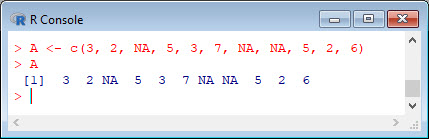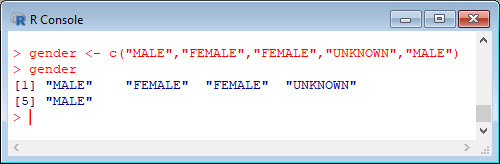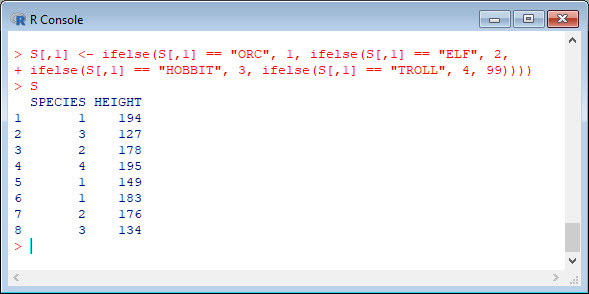You can re-code an entire vector or array at once. To illustrate, let’s set up a vector that has missing values.
```A <- c(3, 2, NA, 5, 3, 7, NA, NA, 5, 2, 6) A```We can re-code all missing values by another number (such as zero) as follows:
```A[ is.na(A) ] <- 0 A```Let’s re-code all values less than 5 to the value 99.
```A[ A < 5 ] <- 99 A```However, some re-coding tasks are more complex, particularly when you wish to re-code a categorical variable or factor. In such cases, you might want to re-code an array with character elements to numeric elements.
```gender <- c("MALE","FEMALE","FEMALE","UNKNOWN","MALE") gender```Let’s re-code males as 1 and females as 2. Very useful is the following re-coding syntax because it works in many practical situations. It involves repeated (nested) use of the `ifelse()` command.
`ifelse(gender == "MALE", 1, ifelse(gender == "FEMALE", 2, 3))`The element with unknown gender was re-coded as 3. Make a note of this syntax. It’s great for re-coding within R programmes.
Another example, this time using a rectangular array.
```A <- data.frame(Gender = c("F", "F", "M", "F", "B", "M", "M"), Height = c(154, 167, 178, 145, 169, 183, 176)) A```We have deliberately introduced an error where gender is misclassified as `B`. This one gets re-coded to the value 99. Note that the `Gender` variable is located in the first column, or `A[ ,1]`.
```A[ ,1] <- ifelse(A[ ,1] == "M", 1, ifelse(A[,1] == "F", 2, 99)) A```You can use the same approach to code as many different levels as you need to. Let’s re-code for four different levels. My last example is drawn from the films of the Lord of the Rings and the Hobbit. The sets where Peter Jackson produced these films are just a short walk from where I live, so the example is relevant for me.
```S <- data.frame(SPECIES = c("ORC", "HOBBIT", "ELF", "TROLL", "ORC", "ORC", "ELF", "HOBBIT"), HEIGHT = c(194, 127, 178, 195, 149, 183, 176, 134)) S```We now use nested `ifelse()` commands to re-code Orcs as 1, Elves as 2, Hobbits as 3, and Trolls as 4.
```S[,1] <- ifelse(S[,1] == "ORC", 1, ifelse(S[,1] == "ELF", 2, ifelse(S[,1] == "HOBBIT", 3, ifelse(S[,1] == "TROLL", 4, 99)))) S```We can recode back to characters just as easily.
```S[,1] <- ifelse(S[,1] == 1, "ORC", ifelse(S[,1] == 2, "ELF", ifelse(S[,1] == 3, "HOBBIT", ifelse(S[,1] == 4, "TROLL", 99)))) S```The general approach is the same as before, but now you have a few additional sets of parentheses. That wasn’t so hard! In Blog 21 I will present another tip for data analysis in R.
See you later!
David

#### Annex: R codes used

```# Set up a vector that has missing values.
A <- c(3, 2, NA, 5, 3, 7, NA, NA, 5, 2, 6)
A

# Re-code all missing values by another number (such as zero).
A[ is.na(A) ] <- 0
A

# Re-code all values less than 5 to the value 99.
A[ A < 5 ] <- 99
A

# Re-code an array with character elements to numeric elements.
gender <- c("MALE","FEMALE","FEMALE","UNKNOWN","MALE")
gender

# Re-code males as 1 and females as 2. It involves repeated (nested) use of the ifelse() command.
ifelse(gender == "MALE", 1, ifelse(gender == "FEMALE", 2, 3))

# Another example, using a rectangular array.
A <- data.frame(Gender = c("F", "F", "M", "F", "B", "M", "M"), Height = c(154, 167, 178, 145, 169, 183, 176))
A

# Re-code misclassified B to the value 99. Gender variable is located in the first column, or A[ ,1].
A[ ,1] <- ifelse(A[ ,1] == "M", 1, ifelse(A[,1] == "F", 2, 99))
A

# Another example from the films of the Lord of the Rings and the Hobbit.
S <- data.frame(SPECIES = c("ORC", "HOBBIT", "ELF", "TROLL", "ORC", "ORC", "ELF", "HOBBIT"), HEIGHT = c(194, 127, 178, 195, 149, 183, 176, 134))
S

# Use nested ifelse commands to re-code Orcs as 1, Elves as 2, Hobbits as 3, and Trolls as 4.
S[,1] <- ifelse(S[,1] == "ORC", 1, ifelse(S[,1] == "ELF", 2, ifelse(S[,1] == "HOBBIT", 3, ifelse(S[,1] == "TROLL", 4, 99))))
S

# Recode back to characters.
S[,1] <- ifelse(S[,1] == 1, "ORC", ifelse(S[,1] == 2, "ELF", ifelse(S[,1] == 3, "HOBBIT", ifelse(S[,1] == 4, "TROLL", 99))))
S
```